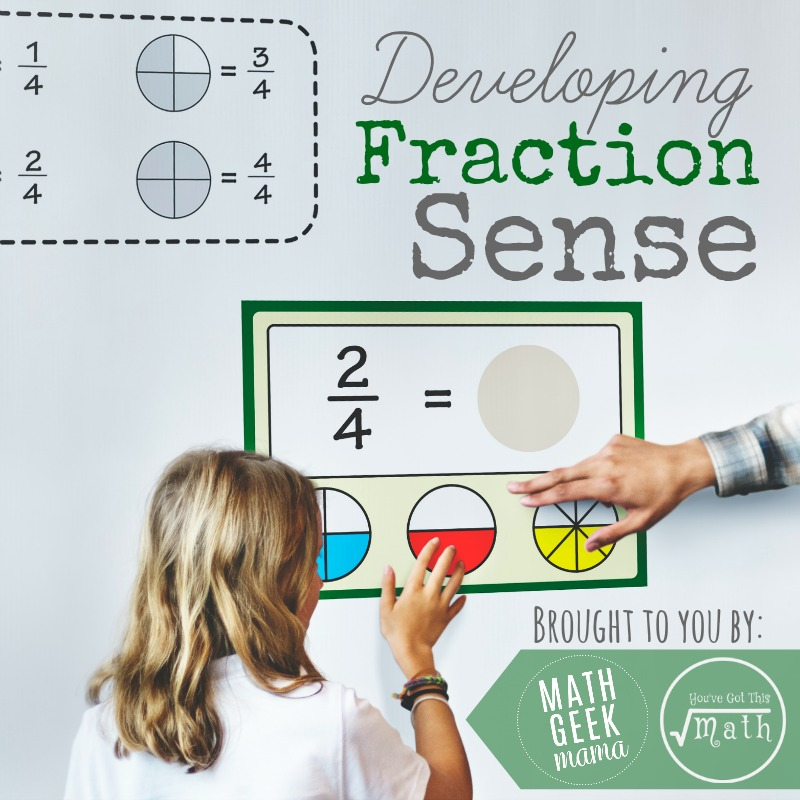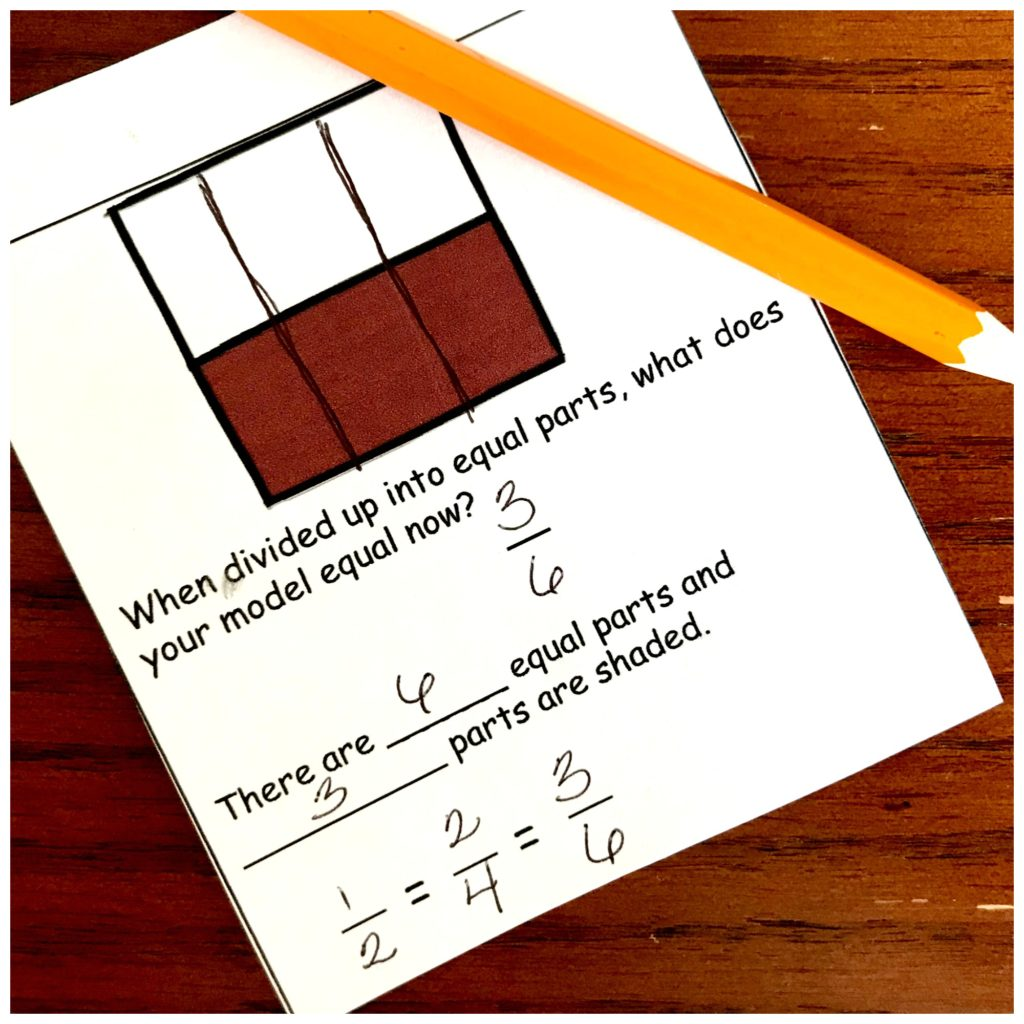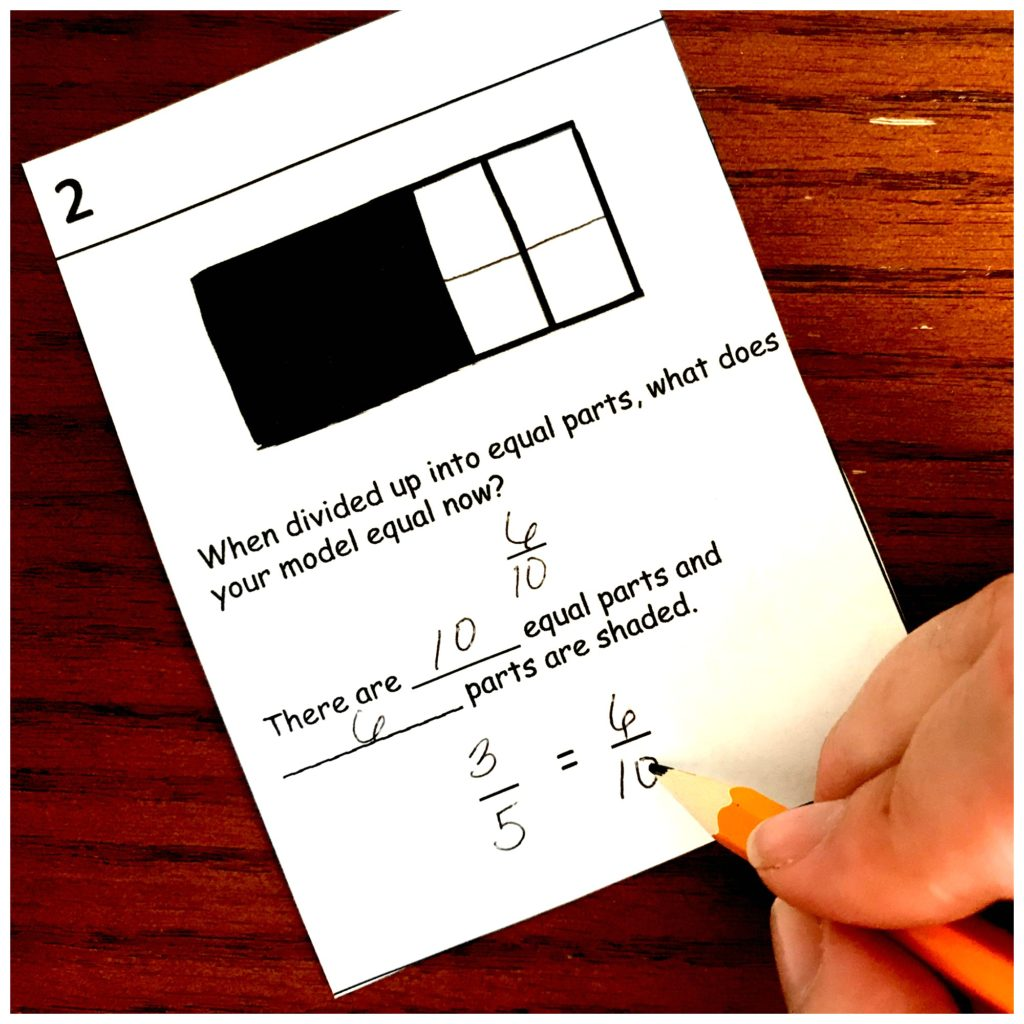Home » Math » Teaching Equivalent Fractions with an Interactive Notebook

Teaching Equivalent Fractions with an Interactive Notebook

This free equivalent fractions interactive notebook is a great way to teach equivalent fractions to your children with visual models or an area model.I love games. I love the way my children get excited when a game comes out, but direct instruction is important too.

One way that I have found to get in direct instruction is to use an interactive notebook! The children are cutting, pasting, and coloring while working out problems. You are getting in some important instructions, and they are having fun.  And when you are finished with the lesson, the children have “notes” on what you just taught.

Today, I’m providing an interactive notebook for equivalent fractions. Using this printable you can help your children understand equivalent fractions using models and number lines.

Before the Lesson

If you are unsure of how to teach modeling equivalent fractions, I highly suggest you hop over to Learn Zillion and see their great explanations. You can also check out this series that Math Geek Mama and I did together. It has lots of great information on how to develop fraction sense using visual models.Once you feel comfortable with this concept all you need to do is gather up a few items.

1. colored pencils

How to Use Equivalent Fractions Interactive Notebook

Step One

First, pass out the first sheet with the square showing 1/2. Each student will need to cut out the four squares and glue them into their notebook. They should place the glue on the back of the paper above the dotted line, and should be placed in order with 1 on the top and 4 on the bottom.

Step Two

Once that part is done, have the students identify the fraction and write it on the fraction line. Next, have them fill in the blanks describing their fraction. Since this concept has been covered when you introduced fractions and reviewed during your mini-lessons they shouldn’t have any trouble filling in the blanks. Right? If someone does struggle, use this as a chance to review the concept of fractions with the student. You may even want to have a manipulative nearby to help.

Step Three

Next, have them move onto the second box. Remind them what you taught during the mini-lesson and how that all fractions must have equal parts. Allow the students to divide up the box any way they choose, as long as each part is still equal. Share the different ways students divided up the box, and even ask questions about each one. Does this work? Why or why not? Are all the parts equal? What will the denominator be? What will the numerator be?

After their boxes are dived, have the students write down the equivalent fraction they made, fill in about the parts of their fraction, and at the end write what the two equivalent fractions are.Continue this routine for box three and four. Stress that the denominator must be different than the first two they just did. By the time they hit the fourth box they should be able to write out three fractions that equal 1/2.Step 4

Work through the other three pages in the same way. By the time they finish, they should have deepened their knowledge of fractions as well as how to find equivalent fractions.I hope this equivalent fraction interactive notebook helps your students begin to understand what happens when we are finding an equivalent fraction!

You’ve Got This

Rachel

Need other Equivalent Fraction activities? Check out 15 other ways to teach and practice equivalent fractions.